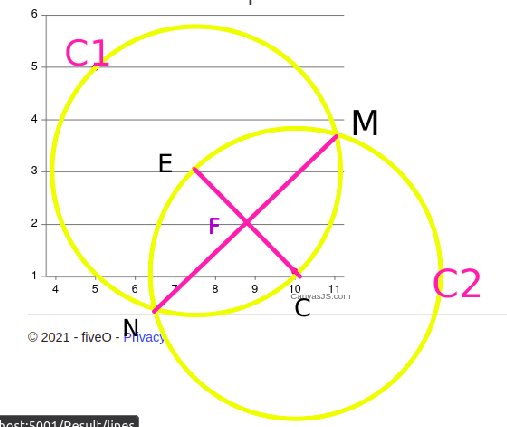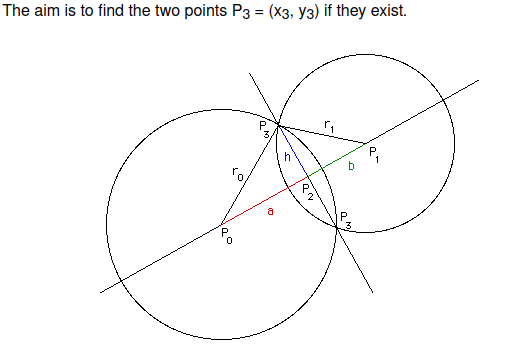•闪存
•博客
• 发言小组
• 投递新闻
• 提问博问
• 添加收藏
•文库

# asking for PointM & PointN coordinates

0悬赏园豆：10 [已解决问题] 解决于 2021-12-18 18:23I am asking for the coordinates of PointM & PointN. The problem is displayed as below figure.Known:

1. C1 is a circle, and C2 is also a circle.
2. EC is the radius of C1 & C2.
3. PointE (Ex,Ey), PointC (Cx, Cy), length of EC

Calculated:
PointF(Fx, Fy),
sEC (slope of line EC),
bEC (y-intercept of line EC),
fMN (slope of line MN)
bMN(y-intercept of line MN)
PointM(x,y) when PointE & PointC are in the horizontal position
PointN(x,y) when PointE & PointC are in the vertical position

I have tried many options to solve the PointM & PointN coordinates when they are in general position, but have not worked so far.

Can anyone help? Java/C/C++/C#/VB/Python/pseudocode is fine.
Many Thanks!

0

Based on Paul,
http://paulbourke.net/geometry/circlesphere/

x3 = x2 +- h ( y1 - y0 ) / d

y3 = y2 -+ h ( x1 - x0 ) / dChecked & it works.

blogger2020 | 菜鸟二级 |园豆：237 | 2021-12-17 19:37

您需要登录以后才能回答，未注册用户请先注册